Subscribe to our newsletter to receive the latest news and events from TWI:

# SENT Stable Tearing Crack Path Deviation and its Influence on J

Emily Hutchison, Philippa Moore and Warren Bath
TWI Ltd, Granta Park Great Abington, Cambridge, CB21 6AL, UK

Paper presented at Proceedings of the ASME 2015 Pressure Vessels & Piping Conference PVP2015, July 19-23, 2015, Boston, Massachusetts, USA

## Abstract

Crack path deviation in Single Edge Notch Tension (SENT) specimens, and its influence on the determination of J, has been investigated as part of the development of a new British Standard for SENT testing, BS 8571 . Crack path deviation by angles up to 50° have been observed during stable tearing in parent material SENT specimens. This paper investigates the effect of crack path deviation on the measured fracture toughness, and offers a correction formula when crack path deviation invalidates the default standard J equations. Mixed mode effects in crack path deviation are also investigated.

A parametric study using finite element analysis has been carried out to compare the value of J calculated using standard equations (which assume a straight crack propagation path) with the value of J calculated using the contour integral method for different levels of crack path deviation. Crack path deviation from the initial crack plane resulted in a non-conservative estimate of fracture toughness using the standard equations. This means that any SENT test exhibiting crack path deviation may need to be discarded, wasting valuable test specimens. Instead, a correction factor has been developed to adjust the calculated value of J if path deviation is observed.

## 1. Introduction

### 1.1. Single edge notch tension (SENT) fracture toughness testing

The fracture toughness of a material is an important input to engineering critical assessment (ECA). When assessing the acceptability of flaws in structures, using standards such as BS 7910:2013 , the fracture toughness of a material is compared to the crack driving force in the structure for relevant in-service loading conditions. If the crack driving force is larger than the fracture toughness of a material, the assessment will deem the structure no longer safe to operate in its current condition. This may require time consuming and costly repairs or replacement. It is therefore important that the fracture toughness used in the assessment is conservative to ensure that a reasonable safety factor is used in the calculation. However, it is also important that the fracture toughness is not over-conservative, as this imposes large safety factors in assessments which may not be necessary.

There are a number of different test specimens which can be used to determine the fracture toughness of a material. Single edge notch tension (SENT) specimens apply tensile loading to a rectangular, pre-notched specimen. Single edge notch bending (SENB) specimens apply bending loading to a similar, rectangular, pre-notched specimen. Compact tension (CT) specimens are designed to be high constraint, despite being loaded in tension, but are more economic in material. They tend to give lower toughness results than both SENT and SENB specimens, and have high machining costs. SENB specimens are currently used most widely for determining conservative fracture toughness measurements of materials for ECA applications.

SENT specimens can be used instead of SENB specimens for determining a more representative and potentially less conservative value of fracture toughness for pipelines. For the same notch depth ratio, the tensile loading used in SENT specimens generates lower constraint at the crack tip than the bending loading in SENB specimens. Therefore, SENB specimens tend to measure lower values of fracture toughness than SENTs. The lower constraint found in SENT specimens is more representative of the constraint found in pipelines, while still giving conservative measurements of fracture toughness. So SENT tests are becoming more favoured in the pipeline industry as a reliable way to determine a conservative value of fracture toughness without the large safety factors involved in using SENB specimens.

Although SENB testing has been standardized for some time (e.g. BS 7448-1:1991 ), there has been no fracture toughness testing standard for SENT specimens until recently. Most guidance on SENT testing methods for industry has been taken from DNV-RP-F108:2006  for multiple-specimen J R-curves. But with SENT testing being carried out more frequently, it is important to standardize the fracture toughness testing method in order for repeatable, reliable results to be obtained from the tests.

A test standard for SENT testing has recently been issued by British Standards as BS 8571:2014 . This is a general test standard, where the fracture toughness can be determined using single-point testing or R-curves (both using single or multiple specimen techniques). The work presented in this paper has been carried out to support the recommendations made to BSI for the development of this standard in future revisions.

### 1.2. Crack path deviation

Crack path deviation (CPD, ie crack propagation at an angle to the original crack plane) has been observed by the authors in parent material SENT specimens. It has also been noted as a problem when testing girth weld heat affected zones (HAZs) and weld metals . In the example shown in Figure 1 the new crack propagation plane during stable tearing was found to deviate from the original fatigue crack plane by an angle of up to 50°. Under such conditions it is important that the value of J output from the test (which assumes a straight crack) is still reliable. In particular, non-conservative measurements (overestimates) of fracture toughness need to be corrected in order to ensure that safe ECAs are carried out, and so that the test specimen is not wasted.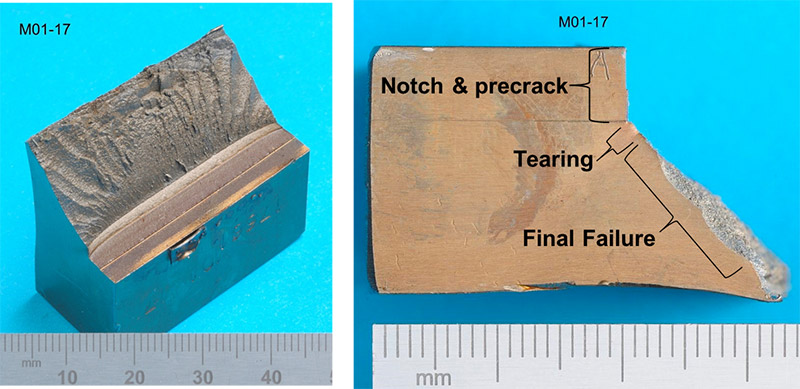Figure 1 crack path deviation of 50° in sent specimen

It is possible that the ductile tearing in Figure 1 has occurred along a path of maximum shear stress/strain under tension, initiated by material inhomogeneity in that direction. In a uniaxial tensile test, the maximum shear planes occur at 45° to the applied load. (It is this which gives rise to the “cup and cone” shape failure in tensile tests.) The orientation of these shear planes seems to influence the direction of ductile crack extension.
CPD in SENT specimens may also result in mixed mode crack tearing. It is possible that the tension orientation has allowed a higher toughness mode of cracking to occur. The tensile loading is no longer acting perpendicular to the crack front, but at a deviated angle, which means that there is now a contribution to in-plane shear (mode II) as well as crack opening (mode I). It is assumed that mode I toughnesses are lower than mixed mode values. Therefore, CPD may result in higher, non-conservative measurement of fracture toughness. This has been further examined using FEA in this paper.

A parametric study has been carried out using finite element analysis (FEA) to compare the value of J calculated using standard equations for experimental determination (which assume a straight crack propagation path) with the value of J calculated using the contour integral method for different levels of crack path deviation. Using these comparisons, a correction factor has been developed to adjust the value of J from the test specimen if path deviation is observed.

## 2. Modelling

### 2.1. Overview

A parametric study has been carried out using FEA of SENT specimens with out-of-plane crack deviation. The models have been used to determine the difference between the critical value of J that would be calculated from an SENT test specimen and the actual value of J in the SENT specimen at failure. The critical J value from an SENT specimen is calculated using the DNV formula  (which is also permitted in BS 8571), which is based on the area under the load-displacement curve from the SENT test. This calculation can be replicated in the FEA models by extracting the load-displacement data and applying the same DNV formula. The actual value of J at the crack tip at the peak load can be calculated from the models using the contour integral method and compared to the J calculated using the DNV formula in order to determine its accuracy.

The effect of two geometric parameters on the value of J from the SENT specimens were investigated: the crack path deviation angle, θ, and the ratio of a0/W where a0 is the initial crack depth (before CPD) and W is the specimen width. These parameters were investigated for a typical pipe material and a fixed crack tearing depth (see Figure 2).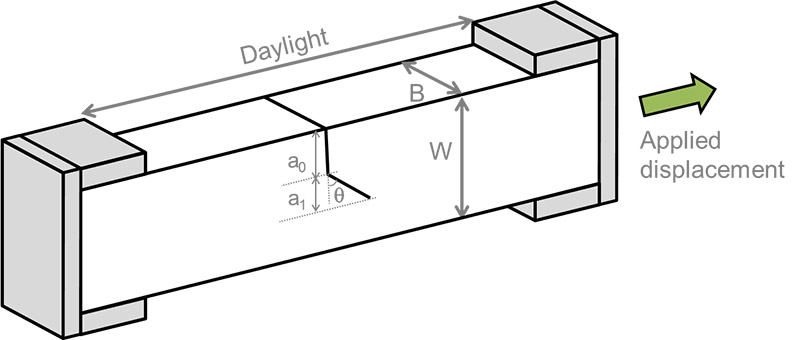Figure 2 SENT model geometry

All models were generated using version 6.13-1 of the pre-processing finite element software Abaqus/CAE and the analyses solved using version 6.13-1 of Abaqus/Standard.

### 2.2. Geometry

A BxB SENT geometry was modelled, with B=W=14mm. These are the same specimen dimensions as some test specimens where path deviation was observed (with a/W=0.4). The SENT specimens were modelled using 2D plane strain elements. This is a reasonable assumption because the thickness of the test specimens are chosen in order to achieve close to plane strain conditions in the centre, where the critical J is measured.

Thirty different specimen geometries were considered in the parametric study. Three different a0/W ratios of 0.3, 0.4 and 0.5 were modelled, with 10 different angles (0°, 3°, 5°, 7°, 10°, 12°, 20°, 30°, 40° and 50°)  at each a0/W ratio. All other geometry parameters were fixed, such as the crack tearing depth, a1, specimen thickness, B, specimen width, W, and the daylight between the grips (as illustrated in Figure 2). The perpendicular length of tearing (a1 in Figure 2) was fixed at 1.5mm, as this was typical of the amount of stable tearing in an SENT test.

A blunt crack tip with 0.01mm radius was modelled to aid convergence, since nonlinear geometry was included in the model. Nonlinear geometry was required in order to calculate the maximum point in the load-CMOD (Crack Mouth Opening Displacement) curve from the SENT specimen. This was important because the maximum point in the Load-CMOD is the point at which the critical value of J is calculated. Although tearing was observed in the real sample, a stationary crack was modelled which included both the initial crack depth (a0) and tearing crack depth (a1) at an angle (θ).

The model was meshed using quadratic, quadrilateral plane strain elements (type CPE8R in Abaqus), with approximately 5,000 elements per model (see Figure 3). A fine, focussed mesh was employed near the crack tip, where the highest stress concentration was expected. The focused mesh had multiple rings of elements around the crack tip to create paths for the calculation of J using contour integrals. A coarser mesh was employed away from the crack tip to reduce computation time.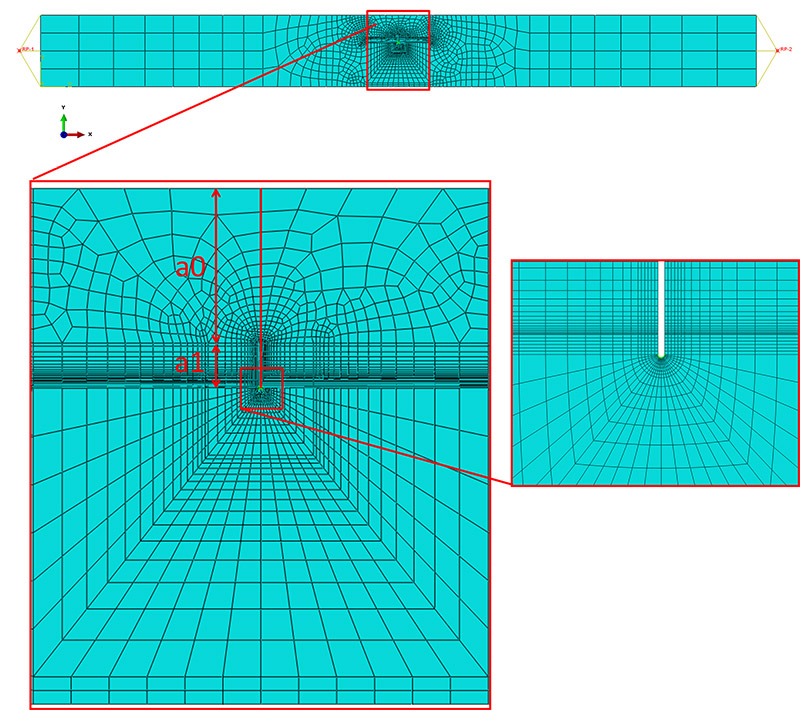Figure 3 straight crack meesh for a0/w=0.3

A condensed mesh was required above the crack tip in order to calculate a path-independent value of J. The formulation of J is only valid over a straight crack path. Therefore, the value of J could only be calculated accurately between the crack tip and the ‘kink’ in the crack (i.e. along the length of a1 in Figure 2). This made it difficult to ensure that path-independence had been achieved in the calculation of the J contour integral, particularly because nonlinear geometry was employed. Therefore, the focussed mesh was made as large as possible below the crack in order to include a large area of the specimen within the contour integral (see Figure 3). This made path-independence of J easier to achieve. It was necessary to have the same number of elements between the crack tip and the ‘kink’ as the number of elements below the crack tip. This was in order to create paths for the J contour integral around the crack tip which included as much of the specimen as possible, whilst not including the crack ‘kink’, where the value of J is not well defined.

The models were generated in two separate stages. Firstly, the SENT model was generated with a straight crack front (see Figure 3), with the correct initial crack depth and tearing crack depth. The crack tearing depth, a1, was modelled as straight, with θ=0° at this stage. This allowed a suitable, structured mesh to be generated around the crack. Secondly, the nodal coordinates of the mesh were transformed in order to introduce the crack path deviation angle, θ, over the tearing depth, a1. The nodal coordinates were shifted using a weighted function around the crack tip ‘box’ (see Figure 4), which applied a shear in the x-direction, which varied bi-linearly over the crack tip box. This meant that the nodal coordinates were shifted most at the centre of the ‘crack box’, and least at the edge of the ‘crack box’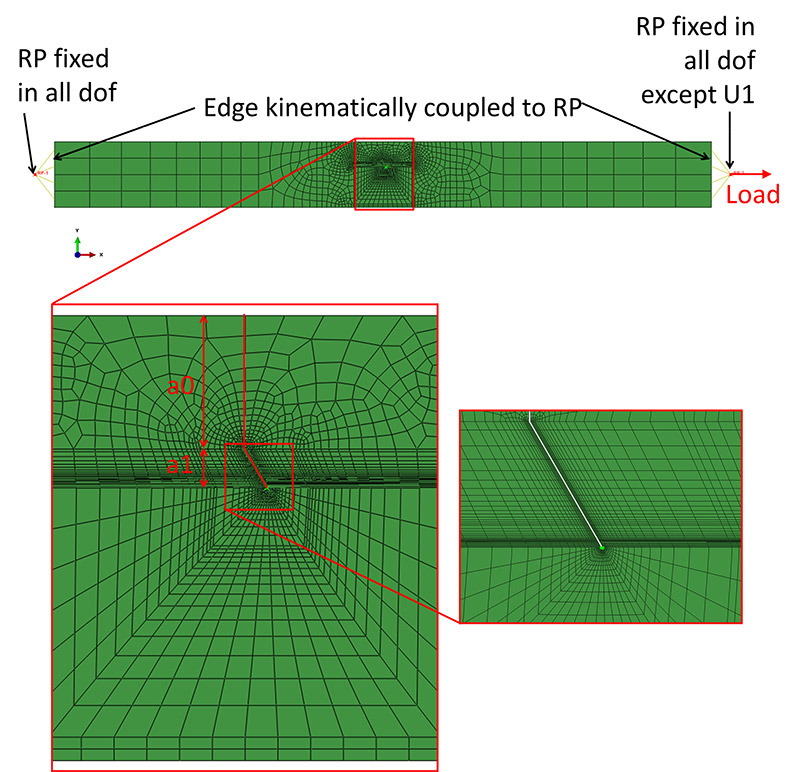Figure 4 deviated crack mesh for a0/w=0.3, θ=30°

### 2.3. Materials

The out-of-plane tearing was observed in parent material SENT specimens. The material properties for the FEA were therefore taken from parent material experimental data after being smoothed and averaged. The engineering stress strain curve is shown in Figure 5. This was converted to true stress and true plastic strain, and the true stress was assumed to be constant once it had reached its maximum in the test. This was input as tabulated true stress, true plastic strain data into Abaqus. The elastic properties of steel were assumed with Young’s Modulus of 207GPa and Poisson’s ratio of 0.3.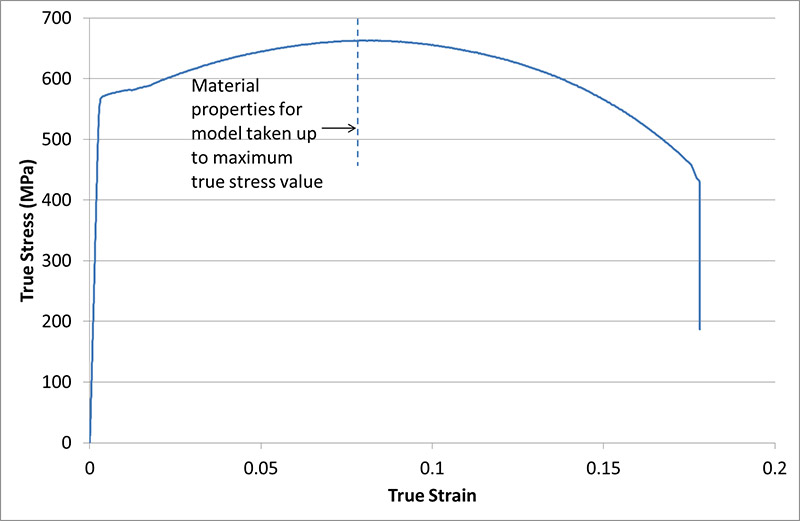Figure 5 engineering averaged and smoothed stress strain curve from tensile data

### 2.4. Loads and Boundary Conditions

The load was applied using clamps at the ends of the specimen. Therefore, the ends of the model specimen were kinematically coupled to reference points in order to apply displacement boundary conditions (see Figure 4). The trailing end (in the –ve x-direction) was fixed in all degrees of freedom. The displacement load was applied to the leading end (5mm) in the +ve x-direction, with all other degrees of freedom fixed to simulate the clamped conditions. This arbitrary, large displacement was chosen in order to ensure that the maximum point in the load-CMOD curve was reached in the simulation.

### 2.5. Outputs

The crack driving force, J, was calculated using two different methods: the contour integral method and the DNV method.

The J-integral was calculated in the models using a domain integral technique over many contours. Convergence was achieved before reaching the ‘kink’ in the crack path, so that the definition of J was still valid. This was achieved by including a large specimen area in the calculation.

The J-integral was also calculated using the equations that would be used for a real SENT test specimen. The equation given in DNV RP F108:2008 is given in Equation 1.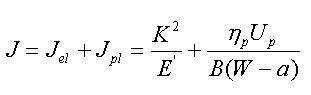Where:

Jel is the elastic component of J,
Jpl is the plastic component of J,
E’ is the longitudinal elastic modulus in plane strain, equal to E/(1-ν2)
ηp is a dimensionless function of geometry and material,
Up is the plastic part of the load versus CMOD curve,
B is the specimen thickness,
W is the specimen width,
a is the initial crack length,
(W-a) is the remaining ligament, also known as b

Most terms are calculated using geometry and materials parameters, apart from K and Up (the detailed equations and method are provided in DNV RP F108 ). The stress intensity factor, K, is calculated using the maximum load reached during the test as well as various geometry parameters. The Up term is calculated using the plastic area under the load-CMOD curve up to maximum load. The value of J according to DNV was therefore calculated using the load-CMOD curve output from the model. Note that in Equation 1 the value of J is calculated using the initial crack depth, a0, because this is the crack depth known at the start of the experiment. It is not reasonable to calculate the DNV equations using the crack depth, a0, when comparing them to FEA because the stationary crack in the model actually had initial depth a0 + a1. The J-integral calculated using the contour integral method also takes into account the total crack depth (a0 + a1). Therefore, for a direct comparison between the contour integral method and the DNV method of calculating J, the crack depth was taken as a0 + a1

## 3. Results

The J-integral calculated using the contour integral method (i.e. the actual value of J at the crack tip) was compared to the DNV calculated value of J (i.e. the value that would be measured in the real SENT experiment). DNV uses initial crack length to calculate J (see Equation 1). But the BS 8571 committee is considering J equations which include tearing length in J determination. For validation, therefore, it is consistent to use the DNV J equation (Equation 1) but including the crack tearing length in comparison with the J from the contour integral method.

### 3.1. Parametric Study

The parametric study has shown that an SENT specimen which exhibits significant out-of-plane tearing will result in a non-conservative measurement of fracture toughness. This is demonstrated in Figure 6, where the value of J is compared from FEA (contour integral method) and from DNV (standard equation) for different crack path deviation angles for a0/W=0.3. The values of J from DNV and FEA at 0° deviation agree very well, and continue to agree within 10% up to an angle of approximately 25°. As the crack path deviation angle increases the value of J calculated from FEA decreases, whereas J from DNV remains approximately constant. This means that the toughness predicted from DNV is over-predicted and non-conservative compared to the actual value of J at the crack tip calculated from FEA. At the steepest angle of tearing (50°), the value of J from FEA was over-estimated by approximately 54%.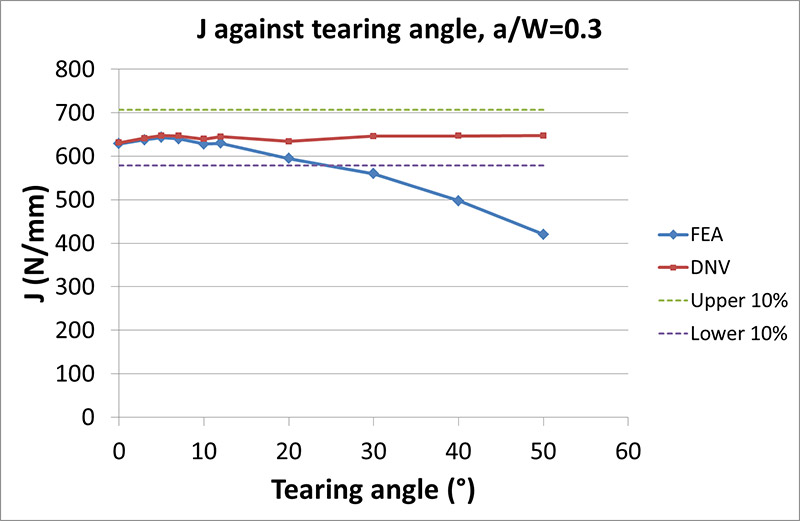Figure 6 J from FEA compared to DNV against tearing angle for a0/w=0.3

The value of J from DNV remains approximately constant because the crack depth is the same even though the deviation angle varies. The geometry parameters considered in the DNV equations are the same for each of the cases even when the crack path deviation angle is varied (see Figure 7). The only parameter which changes in the DNV equation is the plastic area under the load-CMOD curve, which also remains approximately constant across all the cases (see Figure 8). This means that the FEA shows that the energy of the overall system does not change significantly for a deviating crack compared to a straight crack. Although this result was not anticipated, it can be explained by considering the stiffness of the specimen. The overall stiffness of the system is related to the projected cross sectional area of the crack (i.e. the crack depth), which does not change with CPD (see Figure 7).  Therefore, the overall energy in the system, which is related to the stiffness, does not change.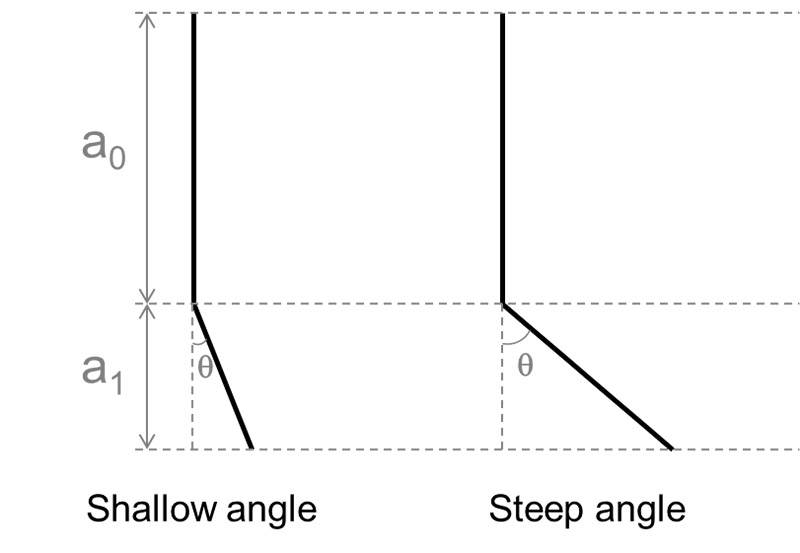Figure 7 crack parameters with different crack path deviation angles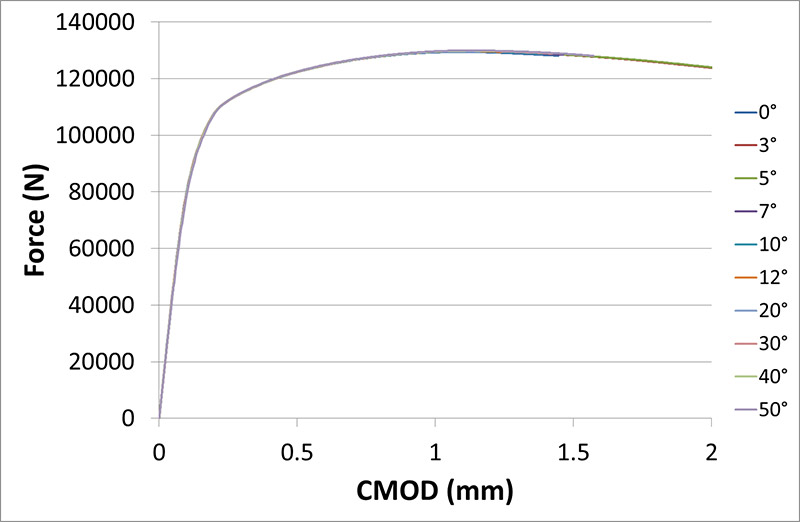Figure 8 load against crack mouth opening displacement (CMOD) for different tearing angles, with a0/w=0.3. the curves are all effectively superposed

The value of J from FEA decreases as the angle increases (see Figure 6), which is due to the increasing overall crack length. The crack depth (a0 + a1) ­remained the same in all the analyses. (This was the parameter input into the DNV equations, which would be characterized from the photograph of the fracture surface after the test, as the crack shape in the perpendicular plane.) But the overall crack length increased, because of the deviation angle over the tearing length, a1. The overall crack tearing length increases from a1 to a1/cosθ, which increases as θ increases. This is discussed further in section 3.2.

Similar trends were observed independent of the a/W ratio, where the value of J from FEA decreased with increasing deviation angle. This is shown in Figures 6, 9 and 10. The value of J for a straight crack front decreases with increasing a0/W ratio, as would be expected due to the higher constraint in the specimen. For each a0/W ratio, the DNV value of J remains approximately constant at the same value as calculated for the straight crack. However, the value of J from FEA decreases with increasing crack path deviation angle for a0/W of 0.3, 0.4 and 0.5. DNV calculations overestimate J at the largest deviation angle of 50° by a similar percentage across all a0/W ratios (51% for a0/W of 0.4 and 55% for a0/W of 0.5). The angle at which 10% deviation occurs between DNV and FEA also remains approximately constant at 25° independent of a0/W ratio (27° for a0/W of 0.4 and 25° for a0/W of 0.5). Therefore, if the crack path deviation is at an angle of less than 25° then the existing J equations can be considered sufficiently accurate without modification, being within 10% error. If the angle of path deviation is greater than 25° then the value of J should be corrected to avoid excessive non-conservatism.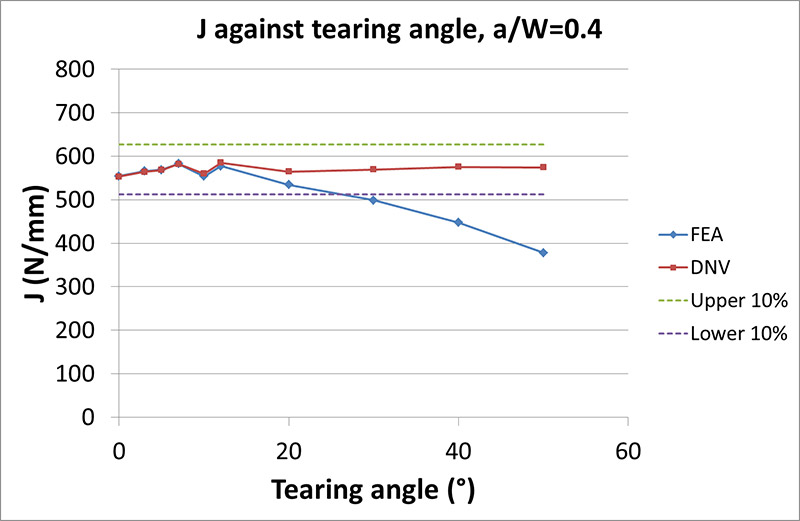Figure 9 J from FEA compared to DNV against tearing angle for a0/w=0.4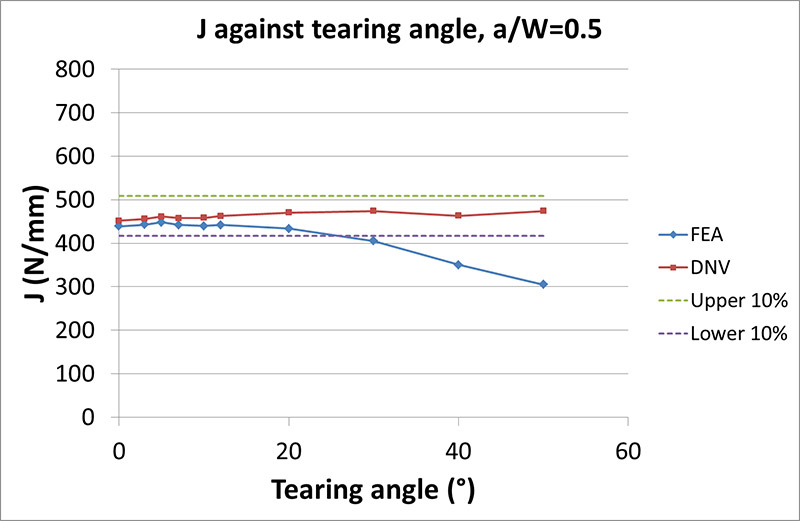Figure 10 J from FEA compared to DNV against tearing angle for a0/w=0.5

### 3.2. Path deviation correction formula

The results from the parametric study enabled a general correction formula for path deviation of SENT specimens to be developed. J can be calculated for SENT specimens using Equation 2, where B is the specimen thickness, U is the strain energy and a is the crack length. The strain energy, U, can be calculated using the Equation 3, where P is the tensile load applied to the specimen, and δ is the applied displacement.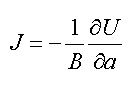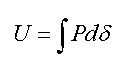The strain energy, U, can be approximated using the area under the load against CMOD curve. As noted in the FEA results, the load-CMOD curve is not significantly affected by crack path deviation compared to a straight crack. Therefore, Equation 4 can be deduced. However, the value of J as the crack path deviation angle varies is not constant (see Equation 5, where ΔaT is the total crack length).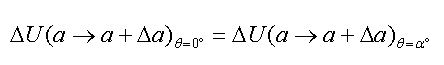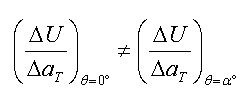The change in total crack length (ΔaT) can be calculated using Equation 6.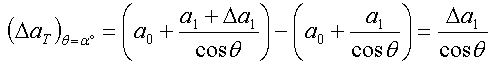Given Equations 7, 8 and 9 (where avert is the vertical component of crack depth), it is then possible to derive a relationship between the strain energy derivative in the straight crack front and the deviated crack front (Equation 10). Therefore, for the same specimen thickness, it is possible to relate J from a straight crack front to J from a deviated crack front using Equation 11.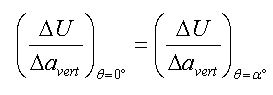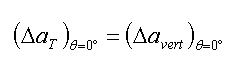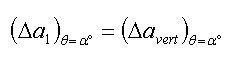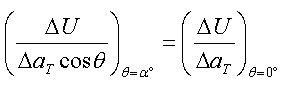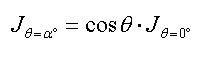This simple correction (Equation 11) can be applied to the results derived from FEA by multiplying the J calculated from DNV by cosθ, where θ is the angle of crack path deviation. The results are shown in Figure 11. The deviated crack values of J from FEA agree with the J calculated from the correction formula to within 3%. These differences are probably due to slight mesh sensitivity in the results. The equation shows that the only difference in the calculation of J comes from the difference in overall crack length, rather than crack depth used in the DNV equations.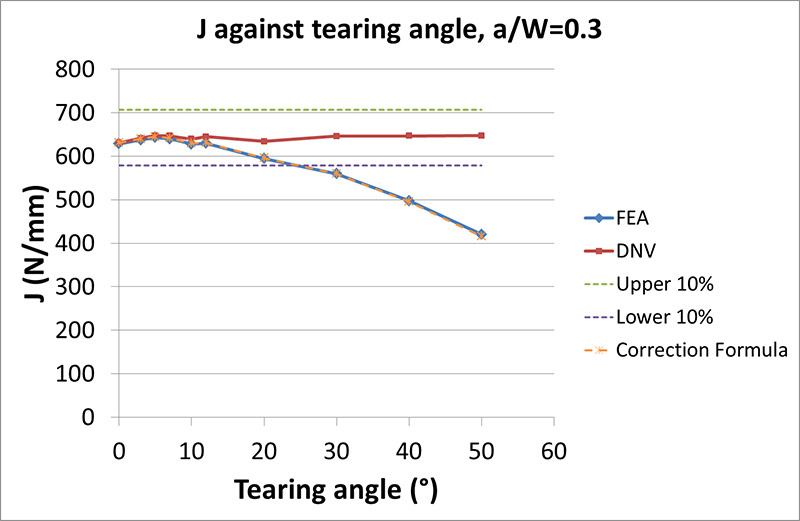Figure 11 J from FEA compared to DNV and the correction formula against tearing angle for a0/w=0.3. the correction formula results lie on the FEA results because of the agreement between the two

The equation was validated by varying a number of other parameters which were not considered in the parametric study. Alternate crack tearing depths (a1) of 0.5mm, 1mm and 2mm were compared with the same geometry. The predicted J values using the correction formula agreed with the FEA results to within 3.5%. Alternative a0/W ratios were also considered, including a0/W=0.45 and 0.55. The equation also remained valid for angles up to 70° from the vertical plane.

### 3.3. Mixed mode effects in CPD

Mixed mode effects were expected as the CPD angle increases, where in-plane shear occurs (mode II) as well as crack opening (mode I). This was investigated by FEA for a0/W=0.3. It was not suitable to extract K from FEA at the maximum load because there is gross plasticity at this load which would not be taken into account by the linear elastic analysis required to determine K. Therefore, a displacement-based ratio of crack opening to in-plane shear was calculated, which is illustrated in Figure 12. Displacements parallel and perpendicular to the crack were extract from two points (A and B, one on each crack flank) at approximately 0.36mm behind the original crack tip. This distance was chosen based on recommendations to extract CTOD from a single point from Fairchild et al . The displacement contributing to mode I crack opening and the displacement contributing to mode II in-plane shear were calculated using Equations 12 and 13, respectively.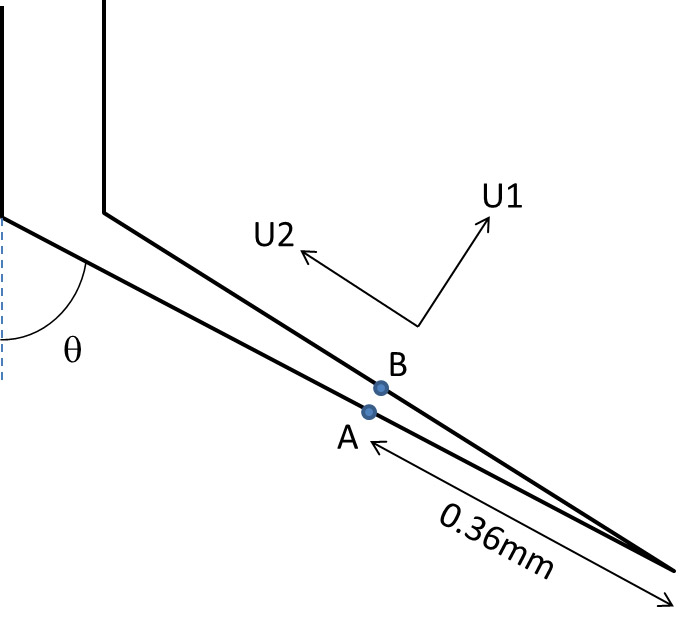Figure 12 displacement-based calculation of mode mixity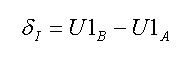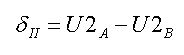There is an approximately linear relationship between the normalized mode mixity and the CPD angle (see Figure 13). For angles up to 50°, the mode mixity can be approximated using Equation 14 below. In other words, for a CPD angle of 0° (i.e. straight crack tearing), purely mode I crack opening is present. As the angle of CPD increases, the mode mixity increases linearly with CPD angle up to an angle of 90° where there is only mode II in-plane shear present.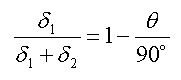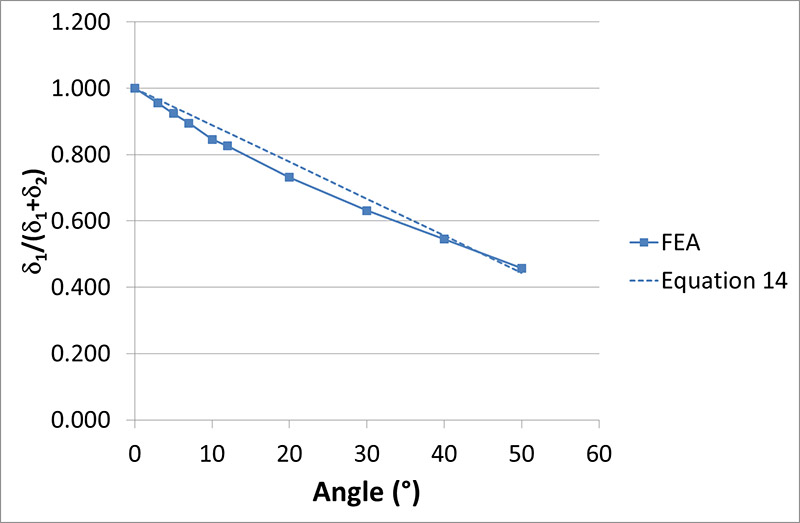Figure 13 normalized mode mixity against CPD angle from FEA compared to linear relationship in equation 14

## 4. Discussion

### 4.1. Application of correction formula

In practical terms, the fracture face can be viewed optically to see whether CPD appears to have occurred. The value of the deviation angle should be determined at the mid-point of the specimen to avoid the influence of any side grooves or shear lips on the measurement. Sectioning at mid-thickness and rough grinding of the specimen should be sufficient to determine the CPD angle (as shown in Figure 1). The correction formula (Equation 11) should then be applied if the CPD angle is above the critical angle of 25° determined in section 3.

Although the phenomenon of CPD has been observed in SENT specimens, to the authors’ knowledge this is the first quantitative investigation. This information would be valuable in order to provide validated guidance to SENT test houses about how to assess its significance. CPD is often unavoidable when it occurs for particular materials, and so rather than simply provide a limit for its validity, this work has sought to provide a simple correction formula to adjust the value of J, when it occurs, based on only the value of J from the test, the crack length measured from the plan view image and the angle of CPD.

In future, when path deviation occurs in SENT test specimens, it can simply be adjusted for rather than cast doubt on the whole validity and value of the data from the test specimen.

## 5. Conclusion

A parametric study using finite element analysis has been carried out to compare the value of J calculated using standard equations (such as DNV-RP-F108 ) with the value of J calculated using the contour integral method for different levels of crack path deviation. The results have shown that:

• If the crack path deviation angle is less than 25° then the existing, standard J equations are sufficiently accurate without modification, being within 10% error.
• If the crack path deviation angle is greater than 25° then the result from the existing, standard J equation should be multiplied by cosθ, where θ is the crack path deviation angle.
• The mode mixity of the stress intensity factor at the crack tip decreases linearly with increasing crack path deviation angle (i.e. pure mode I for θ=0° and pure mode II for θ=90°).

## 6. Acknowledgments

Many thanks to Simon Smith, Tyler London and Henryk Pisarski for their advice and guidance on this paper.

## 7. References

1. BS 8571, 2014: ‘Method of test for determination of fracture toughness in metallic materials using single edge notched tension (SENT) specimens’, British Standards Institution.
2. BS 7910, 2013: ‘Guide to methods for assessing the acceptability of flaws in metallic structures’, British Standards Institution.
3. BS 7448-1, 1991: ‘Fracture mechanics toughness tests – Part 1: Method for determination of KIc, critical CTOD and critical J values of metallic materials’, British Standards Institution.
4. DNV-RP-F108, 2006: ‘Recommended practice DNV-RP-F108, Fracture control for pipeline installation methods introducing cyclic plastic strain’, DNV.
5. Pisarski H. G., 2010: ‘Determination of pipe girth weld fracture toughness using SENT specimens’, Proceedings of the 8th International Pipeline Conference, IPC2010, Calgary, Alberta, Canada, September 27-October 1, 2010.
6. Fairchild D. P., Tang H., Safrova S.Y., Cheng W., Crapps J.M., 2014: ‘Updates to ExxonMobil’s Modelling Approach for Tensile Strain Capacity Prediction’, Proceedings of the Twenty-Fourth International Ocean and Polar Engineering Conference (ISOPE), Busan, Korea, June 15-20, 2014.